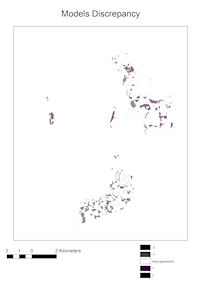## 4.2 Model testing

### 4.2.1 Internal testing

One important aspect to be considered in such a modelling process is the need to test the outcome data. As already mentioned, a predictive model is a statistical hypothesis that needs to be validated in order to assess its level of confidence. Generally, the test of a model should take place through different stages: first, it has to be based on internal data, the same on which the processing has been performed; afterwards, it has to be carried out on new, independent data, which archaeologists collect from the field. In the Pisa coastal plain project, the first stage of testing was carried out by measuring the model performances, which is the degree to which a model correctly predicts the presence or absence of archaeological remains. A high standard of performance, defined by a gain value very close to 1, is based on the calculation of the so-called Kvamme gain, defined by the algorithm:

G = 1- (Pa/Ps) [Verhagen 2007]

where Pa corresponds to the area proportion of the zone of interest and Ps to the proportion of sites found in the zone of interest.

When the final value is close to 1, it indicates a good working model in terms of accuracy and precision. In this case we obtained, both in the 'training' and in the 'testing' model, a very good standard of performance related to the high risk level areas, resulting in a Kvamme`s gain values of 0.980 and 0.888. Subsequently, further internal testing was applied to the two models, in order to quantify their difference and discrepancy levels, by means of the following algorithm:

K= (Po – Pe)/(1-Pe) [Verhagen 2007]

where Po is the observed agreement and Pe is the expected agreement between the two classifications. The final value obtained, 0.952, seems to confirm a nearly complete agreement between the two models (Figure 4).

Figure 4: Discrepancy map obtained by comparing the two training and testing modelsFinally, as for every statistical hypothesis, it is important to test it by verifying the discrepancy between a starting assumption and the available data, where we consider as available data the dataset made by new, independent elements not used for building the model (Verhagen 2007). In this sense, statistics help us in answering the archaeological questions which led to the creation of the predictive model (Fletcher and Lock 2005), making a quantitative assessment of the level of confidence which archaeologists can look at.